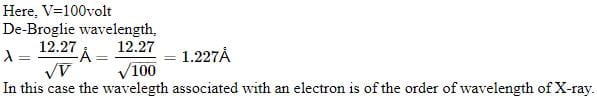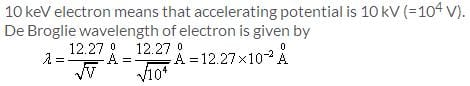NEET  >  Test: Dual Nature of Matter

# Test: Dual Nature of Matter

Test Description

## 10 Questions MCQ Test Physics Class 12 | Test: Dual Nature of Matter

Test: Dual Nature of Matter for NEET 2023 is part of Physics Class 12 preparation. The Test: Dual Nature of Matter questions and answers have been prepared according to the NEET exam syllabus.The Test: Dual Nature of Matter MCQs are made for NEET 2023 Exam. Find important definitions, questions, notes, meanings, examples, exercises, MCQs and online tests for Test: Dual Nature of Matter below.
Solutions of Test: Dual Nature of Matter questions in English are available as part of our Physics Class 12 for NEET & Test: Dual Nature of Matter solutions in Hindi for Physics Class 12 course. Download more important topics, notes, lectures and mock test series for NEET Exam by signing up for free. Attempt Test: Dual Nature of Matter | 10 questions in 10 minutes | Mock test for NEET preparation | Free important questions MCQ to study Physics Class 12 for NEET Exam | Download free PDF with solutions
 1 Crore+ students have signed up on EduRev. Have you?
Test: Dual Nature of Matter - Question 1

### What is the de-Broglie wavelength associated with an electron accelerated through a potential of 100 volts?

Detailed Solution for Test: Dual Nature of Matter - Question 1and as we know 1Å = 0.1 nm, so 1.227Å = 0.122 nm

Test: Dual Nature of Matter - Question 2

### Davisson and Germer experiment proves the

Detailed Solution for Test: Dual Nature of Matter - Question 2

The Davisson-Germer experiment demonstrated the wave nature of the electron, confirming the earlier hypothesis of deBroglie. Putting wave-particle duality on a firm experimental footing, it represented a major step forward in the development of quantum mechanics. The Bragg law for diffraction had been applied to x-ray diffraction, but this was the first application to particle waves.

Test: Dual Nature of Matter - Question 3

### An electron, proton and a jeep all have the same wavelength. The one possessing the highest velocity is​

Detailed Solution for Test: Dual Nature of Matter - Question 3

The de-broglie equation states, mv=h/l

= v=h/lm

the velocity here is inversely dependant on the masses.

Higher the mass lower will be the velocity.

Therefore, electron will have a higher velocity to produce matter waves of same wavelength.

Test: Dual Nature of Matter - Question 4

Objects with mass behave as

Detailed Solution for Test: Dual Nature of Matter - Question 4

We have mass – a particle property, in the same equation as wavelength – a wave property. Thus if matter exhibits wave properties, it must be given by equation (3). Equation (3) is the de Broglie equation and represents the wave-particle duality. Hence we say that everything in the Cosmos exhibits a dual nature.

Test: Dual Nature of Matter - Question 5

An α – particle and a deutron are accelerated through the same potential difference. What will be the ratio of their de-Broglie wavelength?​

Detailed Solution for Test: Dual Nature of Matter - Question 5

Mass of alpha(a)= 4.
mass of deutron(d) =2.
wavelength=h/mv
Potential are same of both particle so their speed will be same .
Here, wavelength is inversely proportional to mass
so,
wavelength of alpha/wavelength of deutron = mass of deutron /mass of alpha.= 2/4=1/2

Test: Dual Nature of Matter - Question 6

Of the following moving with the same momentum, the one which has the largest wavelength is​

Detailed Solution for Test: Dual Nature of Matter - Question 6

De Broglie wavelength = h/mv,
where h = plancks constant, mv = momentum.
As they move with same momentum, de Broglie wavelength remains constant for all.

Test: Dual Nature of Matter - Question 7

The velocity of matter waves are​

Detailed Solution for Test: Dual Nature of Matter - Question 7

The variable v can either be taken to be the speed of the particle or the group velocity of the corresponding matter wave. Since the particle speed for any particle that has mass (according to special relativity), the phase velocity of matter waves always exceeds c, i.e.

Test: Dual Nature of Matter - Question 8

The wavelength of 1 keV photon is

Detailed Solution for Test: Dual Nature of Matter - Question 8

There is a direct relation ,
energy= 12400/wavelength(i e ,lambda in angstroms).
given E= 1k e v = 1000 e.v
wavelength = 12400/1000
wavelength= 124/10
wavelength= 12.4

Test: Dual Nature of Matter - Question 9

The de-Broglie wavelength of an electron is 1.0 nm. What is the retarding potential required to stop it?​

Detailed Solution for Test: Dual Nature of Matter - Question 9

λ=h/P
Here P= √2mk
K=kinetic energy
λ=h/√2mk
By energy conversion
K=eVs
λ=h/√2meVs
√Vs=h/ λ√2me
Vs=h2/ λ2me
Vs=(6.626x10-34)2/(1x10-9)2x2x9.11x10-31xe
Where e=1.602x10-19
Vs=43.9x10-68/29.1x10-68
=1.508v
Vs=1.5v

Test: Dual Nature of Matter - Question 10

The de Broglie wavelength of 10 KeV electron is

Detailed Solution for Test: Dual Nature of Matter - Question 10## Physics Class 12

157 videos|452 docs|213 tests
 Use Code STAYHOME200 and get INR 200 additional OFF Use Coupon Code
Information about Test: Dual Nature of Matter Page
In this test you can find the Exam questions for Test: Dual Nature of Matter solved & explained in the simplest way possible. Besides giving Questions and answers for Test: Dual Nature of Matter, EduRev gives you an ample number of Online tests for practice

## Physics Class 12

157 videos|452 docs|213 tests

### How to Prepare for NEET

Read our guide to prepare for NEET which is created by Toppers & the best Teachers